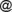# Issue:A note on mean value and dispersion of intuitionistic fuzzy events

 shortcut http://ifigenia.org/wiki/issue:nifs/26/4/1-8
Title of paper: A note on mean value and dispersion of intuitionistic fuzzy events
Author(s):
 Katarína Čunderlíková Mathematical Institute, Slovak Academy of Sciences, Stefanikova 49, 814 73 Bratislava, Slovakia cunderlikova.lendelovagmail.com
Published in: Notes on Intuitionistic Fuzzy Sets, Volume 26 (2020), Number 4, pages 1–8
DOI: https://doi.org/10.7546/nifs.2020.26.4.1-8
Abstract: In this paper, we compare two definitions of mean value and dispersion for intuitionistic fuzzy events. We show the connection between these two definitions and we introduce some types of mean values induced by intuitionistic fuzzy state and by intuitionistic fuzzy probability.
Keywords: Intuitionistic fuzzy event, Intuitionistic fuzzy observable, Intuitionistic fuzzy state, Intuitionistic fuzzy probability, Intuitionistic fuzzy mean value, Intuitionistic fuzzy dispersion, Intuitionistic fuzzy distribution function.
AMS Classification: 03B52, 60A86, 60E05, 28A35.
References:
1. Atanassov, K. T. (2016). Intuitionistic fuzzy sets. VII ITKR Session, Sofia, 20-23 June 1983 (Deposed in Centr. Sci.-Techn. Library of the Bulg. Acad. of Sci., 1697/84) (in Bulgarian). Repr. Int. J. Bioautomation, 20, S1–S6.
2. Atanassov, K. T. (1999). Intuitionistic Fuzzy Sets: Theory and Applications, Physica Verlag, New York.
3. Atanassov, K. T. (2012). On Intuitionistic Fuzzy Sets, Springer, Berlin.
4. Bartkova, R., & Čunderlíková, K. (2018). About Fisher–Tippett–Gnedenko Theorem for Intuitionistic Fuzzy Events. Advances in Fuzzy Logic and Technology 2017, J. Kacprzyk et al. eds. IWIFSGN 2017, EUSFLAT 2017. Advances in Intelligent Systems and Computing, Vol 641, Springer, Cham, 125–135.
5. Lendelova, K. (2005). Convergence of IF-observables. Issues in the Representation and Processing of Uncertain and Imprecise Information – Fuzzy Sets, Intuitionistic Fuzzy Sets, Generalized nets, and Related Topics, EXIT, Warsawa, 232–240.
6. Lendelova, K. (2006). Strong law of large numbers for IF-events. Proceedings of the Eleventh International Conference IPMU 2006, 2-7 July 2006, Paris, France, 2363–2366.
7. Lendelova, K. (2006). Conditional IF-probability. Advances in Soft Computing: Soft Methods for Integrated Uncertainty Modelling, Vol 37, Springer, Berlin, Heidelberg, 275–283.
8. Lendelova, K., & Riečan, B. (2004). Weak law of large numbers for IF-events. Current Issues in Data and Knowledge Engineering, Bernard De Baets et al. eds., EXIT, Warszawa, 309–314.
9. Riečan, B. (2003). A descriptive definition of the probability on intuitionistic fuzzy sets. EUSFLAT ’2003 (M. Wagenecht, R. Hampet eds.), Zittau-Goerlitz Univ. Appl. Sci., 263–266.
10. Riečan, B. (2004). Representation of Probabilities on IFS Events. Soft Methodology and Random Information Systems (Lopez-Diaz et al. eds.), Springer, Berlin Heidelberg New York, 243–248.
11. Riečan, B. (2005). On the probability on IF-sets and MV-algebras. Notes on Intuitionistic Fuzzy Sets, 11 (6), 21–25.
12. Riečan, B. (2006). On a problem of Radko Mesiar: general form of IF-probabilities. Fuzzy Sets and Systems, Volume 152, 1485–1490.
13. Riečan, B. (2006). On the probability and random variables on IF events. Applied Artificial Intelligence, Proc. 7th FLINS Conf. Genova, D. Ruan et al. eds., 138–145.
14. Riečan, B. (2012). Analysis of fuzzy logic models. Intelligent systems (V. Koleshko ed.), INTECH, 219–244
Citations:

The list of publications, citing this article may be empty or incomplete. If you can provide relevant data, please, write on the talk page.